## 一、股票买卖系列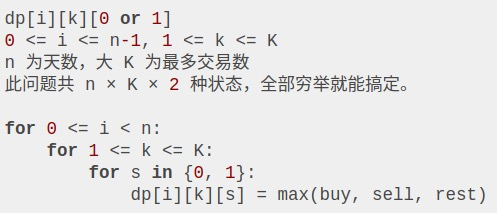dp:今天是第三天，我现在手上持有着股票，至今最多进行 2 次交易。

dp 的含义：今天是第二天，我现在手上没有持有股票，至今最多进行 3 次交易。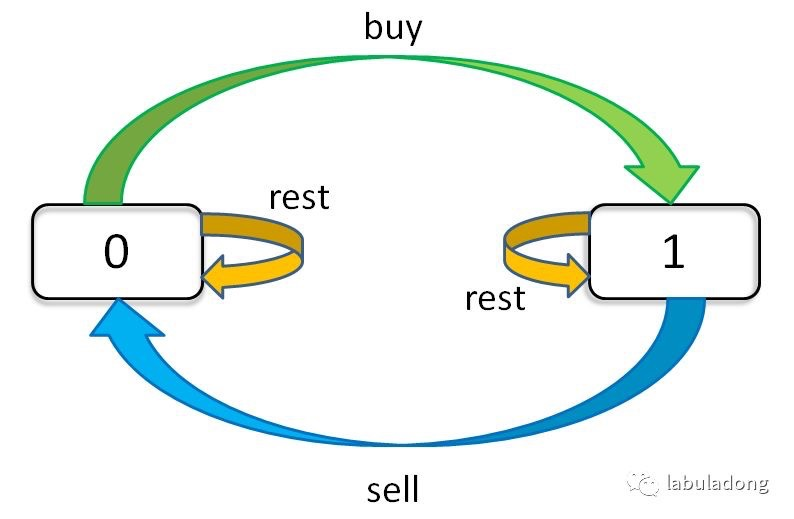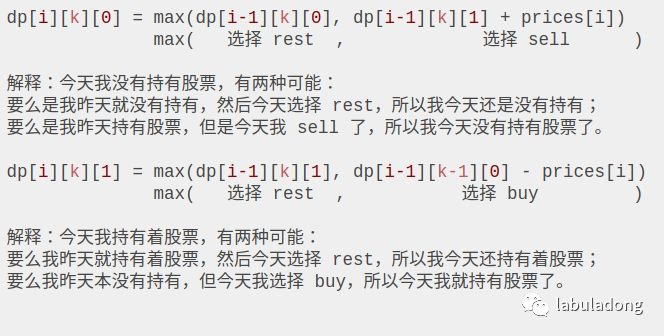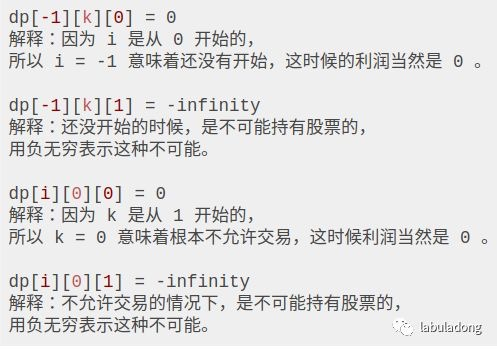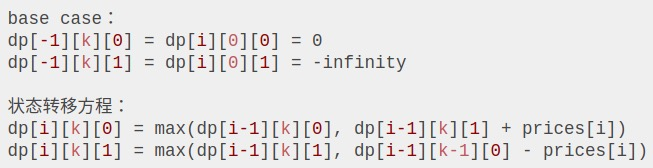### 121. 买卖股票的最佳时机

``````给定一个数组，它的第 i 个元素是一支给定股票第 i 天的价格。

注意利润不能是 7-1 = 6, 因为卖出价格需要大于买入价格；同时，你不能在买入前卖出股票。

``````

K=1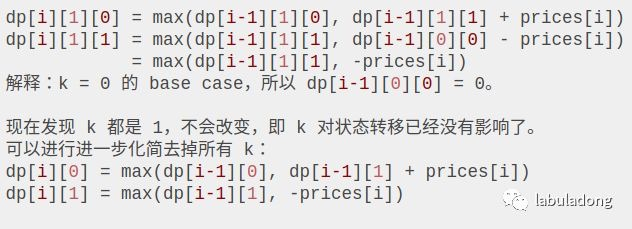### 122. 买卖股票的最佳时机 II

``````给定一个数组，它的第 i 个元素是一支给定股票第 i 天的价格。

随后，在第 4 天（股票价格 = 3）的时候买入，在第 5 天（股票价格 = 6）的时候卖出, 这笔交易所能获得利润 = 6-3 = 3 。

注意你不能在第 1 天和第 2 天接连购买股票，之后再将它们卖出。
因为这样属于同时参与了多笔交易，你必须在再次购买前出售掉之前的股票。

1 <= prices.length <= 3 * 10 ^ 4
0 <= prices[i] <= 10 ^ 4
``````

K=+infinity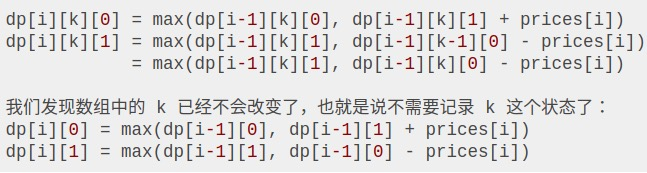### 309. 最佳买卖股票时机含冷冻期

``````给定一个整数数组，其中第 i 个元素代表了第 i 天的股票价格 。​

``````

K=+infinity with cooldown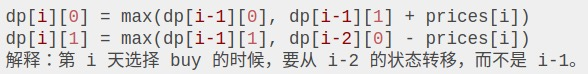### 714. 买卖股票的最佳时机含手续费

``````给定一个整数数组 prices，其中第 i 个元素代表了第 i 天的股票价格 ；非负整数 fee 代表了交易股票的手续费用。

0 < prices.length <= 50000.
0 < prices[i] < 50000.
0 <= fee < 50000.
``````

``````K=+infinity with fee

``````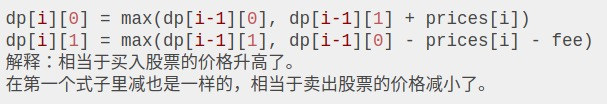### 123. 买卖股票的最佳时机 III

``````给定一个数组，它的第 i 个元素是一支给定的股票在第 i 天的价格。

随后，在第 7 天（股票价格 = 1）的时候买入，在第 8 天 （股票价格 = 4）的时候卖出，这笔交易所能获得利润 = 4-1 = 3 。

注意你不能在第 1 天和第 2 天接连购买股票，之后再将它们卖出。
因为这样属于同时参与了多笔交易，你必须在再次购买前出售掉之前的股票。

``````

``````K=2
``````

k = 2 和前面题目的情况稍微不同，因为上面的情况都和 k 的关系不太大。要么 k 是正无穷，状态转移和 k 没关系了；要么 k = 1，跟 k = 0 这个 base case 挨得近，最后也被消掉了。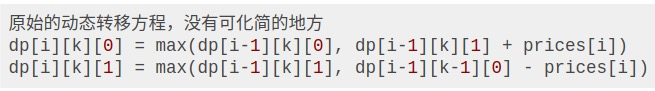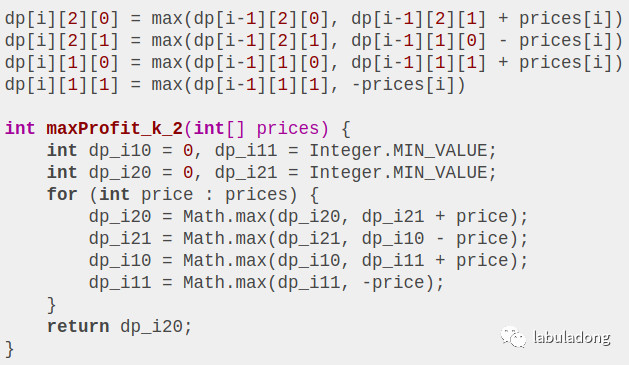### 188. 买卖股票的最佳时机 IV

``````给定一个数组，它的第 i 个元素是一支给定的股票在第 i 天的价格。

随后，在第 5 天 (股票价格 = 0) 的时候买入，在第 6 天 (股票价格 = 3) 的时候卖出, 这笔交易所能获得利润 = 3-0 = 3 。
``````

``````K=any integer
``````